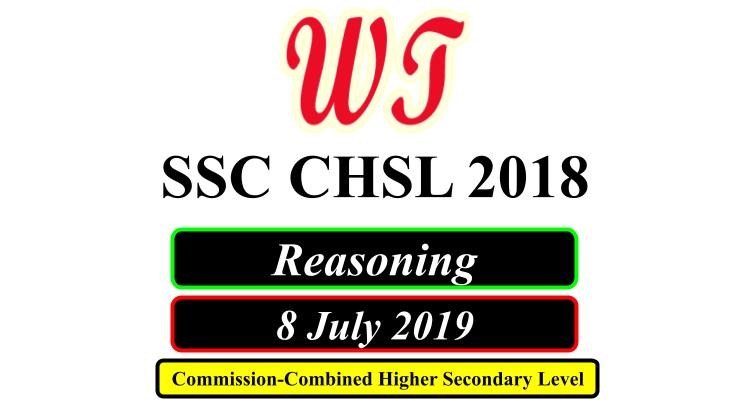Type Here to Get Search Results !

Download SSC ( Staff Selection Commission ) CHSL ( Combined Higher Secondary Level ) Exam, which was held on 8 July 2019, All Reasoning Questions, All Shifts PDF With Answers.

Table of Content

Table of Content (toc)SSC CHSL 8 July 2019 Reasoning Questions PDF Download Free

### SSC CHSL 8 July 2019 Shift 1 Reasoning Questions

Q3:- Select the number-pair in which the two numbers are related in the same way as are the two numbers of the following number-pair.
12 : 102
a.    6 : 52
b.    14 : 126
c.    8 : 68
d.    16 : 218
Q4:- Three of the following four numbers are alike in a certain way and one is different. Pick the number that is different from the rest.
a.    173
b.    193
c.    191
d.    171
Q7:- In a code language, CARNIVAL is written as LPYAJYTG. How will DISTANCE be written as in that language?
a.    RQGBDAMY
b.    RPGBCZLY
c.    RQGBCALY
d.    SQHBCALZ
Q8:- Select the set in which the numbers are related in the same way as are the numbers of the following set.
(8, 27, 64)
a.    (49, 64, 81)
b.    (216, 343, 529)
c.    (343, 512, 729)
d.    (125, 636, 864)
Q9:- Select the option that is related to the third letter-cluster in the same way as the second letter-cluster is related to the first lettercluster.
KSOE : MQQC :: ACZN : ?
a.    CABL
b.    CABK
c.    DACL
d.    CBBL
Q10:- ‘Heart’ is related to ‘Circulation’ in the same way as ‘Lungs’ are related to ‘_________’.
a.    Excretion
b.    Respiration
c.    Oxygen
d.    Ribs

### SSC CHSL 8 July 2019 Shift 2 Reasoning Questions

Q1:- Three of the following four numbers are alike in a certain way and one is different. Pick the number that is different from the rest.
a.    336
b.    224
c.    226
d.    448
Q2:- Arrange the following activities in a logical and meaningful order.
1. Setting up alarm clock
2. Solving question paper
3. Alarm Bell ringing
4. Reach examination center
5. Getting dressed and be ready
a.    3, 1, 5, 4, 2, 6
b.    1, 3, 4, 5, 2, 6
c.    1, 3, 5, 4, 6, 2
d.    1, 3, 5, 4, 2, 6
Q3:- Select the set in which the numbers are related in the same way as are the numbers of the following set.
(8, 39, 5)
a.    (11, 67, 8)
b.    (10, 60, 6)
c.    (9, 55, 4)
d.    (14, 115, 9)
Q4:- Two statements are given, followed by three conclusions numbered I, II and III. Assuming the statements to be true, even if they seem to be at variance with commonly known facts, decide which of the conclusions logically follow(s) from the statements.
Statements:
Some plants are vegetable.
All vegetables are vitamins.
Conclusions:
I. Some vitamins are plants.
II. Some vitamins are vegetables.
III. No vitamin is plant.
a.    Either conclusion I or III follows.
b.    Only conclusions II and III follow.
c.    Only conclusions I and II follow.
d.    Only conclusions I and III follow.

### SSC CHSL 8 July 2019 Shift 3 Reasoning Questions

Q3:- Select the word-pair in which the two words are related in the same way as are the two words in the following word-pair.
Detailed : Meticulous
a.    Hungry : Fulfilled
b.    Loud : Loose
c.    Fat : Obese
d.    Heavy : Light
Q4:- Three of the following four letter-clusters are alike in a certain way and one is different. Pick the odd one out.
a.    RVZD
b.    PTXB
c.    DHLP
d.    JNRW
Q6:- Three of the following four numbers are alike in a certain way and one is different. Pick the number that is different from the rest.
a.    49
b.    64
c.    16
d.    25
Q7:- ‘Tadpole’ is related to ‘Frog’ in the same way as ‘Caterpillar’ is related to ‘_________’.
a.    Baboon
b.    Butterfly
c.    Bat
d.    Boar
Q8:- Select the number-pair in which the two numbers are related in the same way as are the two numbers of the following number-pair. 2 : 9
a.    3 : 26
b.    7 : 50
c.    8 : 65
d.    4 : 65

SSC CHSL Previous Year Paper

SSC CHSL Previous Year English Questions

SSC CHSL Previous Year Reasoning Questions

SSC CHSL Previous Year Aptitude Questions

SSC CHSL Previous Year G.K. Questions